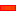﻿ Gears and Four-Bars

# Gears and Four-Bars

## Geared Four-Bar Mechanisms

Geared Four-bar Mechanisms are usually built with:

 • A four-bar mechanism, with at least one Part that rotates continuously
 • One or two Gear-Pairs
 • The input 'drive' gear is fixed to the Coupler, at the distal end of the Crank or Rocker
 • The output gear is usually at the ground joint of the respective Crank or Rocker

'Geared Four-Bar Mechanisms' are often used as 'Function-Generators'. Function-Generators provide an input to output motion relationship.

Typically, the input to these mechanisms is a continuously rotating Crank.

### One Gear-Pair

Regressive and Ir-regressive Gears

I have no idea why they are called 'regressive' and 'ir-regressive' gears!

Regressive gears are at each end of the Crank,

Ir-regressive gears are at each end of the Rocker.

####'Regressive' Geared Four-barSTEP 1: A Four-barSTEP 2: Add a Part to the Ground Point of the Crank

Remember: The Parts must be joined with Pin-Joints to the Points at each end of the Line-of-centres.

Make sure the Point at the end of the new Part is joined to the Crank, and not the Base-Part.

See Select Elements dialog-boxSelect the the CAD-Lines that are in:

 • Coupler as the Input-Part
 • New Part as the Geared-Rocker
 • Crank as the Line-of-centresSTEP 4: Edit the number-of-teeth
 STEP 5: Plot the motion

Add a Measurement FB to measure the motion of the New Geared Rocker.

####'Ir-regressive' Geared Four-barSTEP 1: A Four-barSTEP 2: Add a Part to the ground Point of the Rocker

Remember: The Parts must be joined with Pin-Joints to the Points at each end of the Line-of-centres.

Make sure the Point at the end of the new Part is joined to the Rocker, and not the Base-Part.

See Select Elements dialog-boxSelect the the CAD-Lines that are in:

 • Coupler as the Input-Part
 • New Part as the Geared-Rocker
 • The Rocker as the Line-of-centresSTEP 4: Edit the number-of-teeth
 STEP 5: Edit the length of the Parts.
 STEP 6: Plot the motion

Add a Measurement FB to measure the motion of the New Geared Rocker.

### Two Gear-Pairs

Usually, the two gears on the same shaft at the are edited so that they have the same module and number-of-teeth. Although, this is not necessary.

There is a small complexity in MechDesigner (special rule) that means you must use a 'trick' when you add the second Gear-Pair.

#### Gear-Pair 1STEP 1: A Four-bar

We will add the first Gear-Pair to the Coupler.

The input Gear will be at the end of the Crank.

The motion of the input gear will be the relative motion between the Crank and the Coupler.STEP 2: Add a Part to the end of the Coupler.

Remember: The Parts must be joined with Pin-Joints to the Points at each end of the Line-of-centres.

Make sure the Point at the end of the new Part is joined to the end of the Coupler and not the end of the Rocker.

See Select Elements dialog-boxSelect the:

 • Crank as the Input-Part
 • New Part as the Geared-Rocker (output)
 • The Coupler as the Line-of-centres
 STEP 4: Edit the number-of-teeth.

#### Gear-Pair 2

This step requires a good understanding of the Add Gear-Pair command.STEP 1: Add the Output Part

Make sure it is joined to the Rocker and not the Base-Part.

We want to add the 'Gear-Pair 2'.

The Parts with the Gears must be joined to the end of the Line that will be the Line-of-centres. The Line-of-centres will be the CAD-Line along the Rocker - the output Part of the Four-bar Mechanism.

The Blue Part is joined to the Rocker.

However, the Green Idler Gear Part at the distal end of the Rocker is joined to the Coupler  - not the Rocker.

So, if we tried to add a Gear-Pair, we would not succeed.

We must add a new Part to the distal end of the Rocker

The new Part must:

 • be joined to the distal End of the Rocker.
 • rotate at the same speed as the Idler Gear Part at the distal end of the Rocker - the idler gear.

To do this we:

 • add a New Part to the distal end of the Rocker
 • add a Motion-Dimension FB to specify the position of the new Part relative to the Rocker
 • measure the speed of the Idler Gear with a Measurement FB
 • use the measurement at the output -connector of the Measurement FB as an input to a Motion-Dimension FB to move the new Part.

See Select Elements dialog-boxSTEP 2: Add a new Part

The Parts at each end of the CAD-Line along the Rocker or Blue.

One must be Green.STEP 3: Add a Motion-Dimension FB

The Part is now Green

We must move it at the same speed as the Idler Gear.

To do this we measure the Idler Gear Part relative to the Rocker with a Measurement FB.STEP 4: Add a Measurement FB

Now we can know the motion of the idler relative to the Rocker.STEP 5: Connect the output of the Measurement FB to the input of the Motion-Dimension FB

This makes the Motion-Dimension, and Motion-Part, move with the same motion-values given by the Measurement FB.

The Motion-Part will ave the same motion as the existing Geared Rocker.

Therefore, when we add the new Gear-Pair, the Parts move in the same way.STEP 7: Edit the number-of-teeth so that the 'middle' idler gear has the same number-of-teeth.
 STEP 8: Edit the number-of-teeth for the output gear.STEP 9: Make the Base-Value of the Motion-Dimension FB equal to Zero.

The two Parts at the end of the Rocker are now together.

Also, it makes the Gears appear as Simple Gear-Train.

You may need to edit the Gear Teeth so that the four-bar makes a complete rotation.STEP 10: Measure the motion of the Output Gear.

Tutorial and Reference Help Files for MechDesigner and MotionDesigner 13.2 + © Machine, Mechanism, Motion and Cam Design Software by PSMotion Ltd